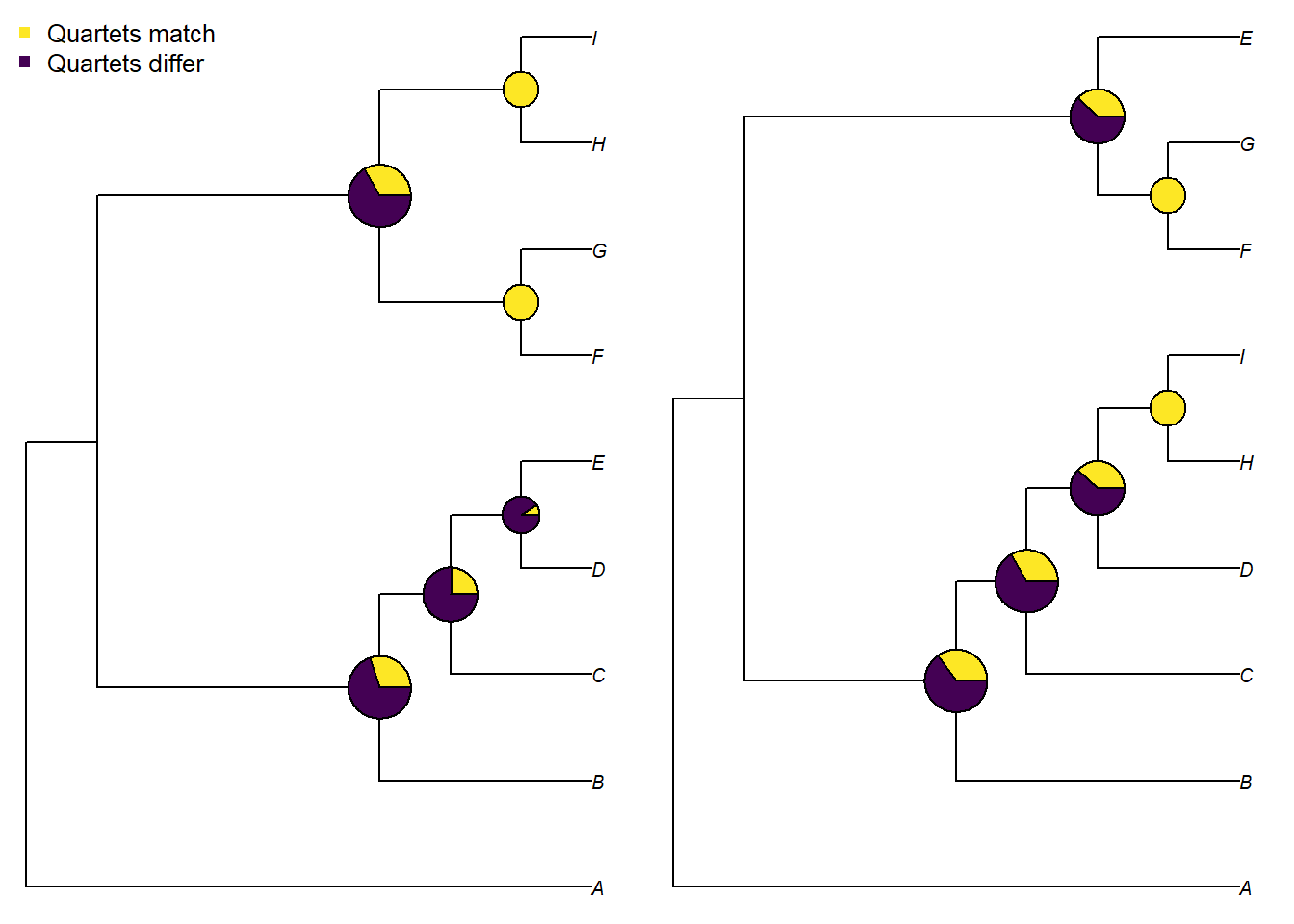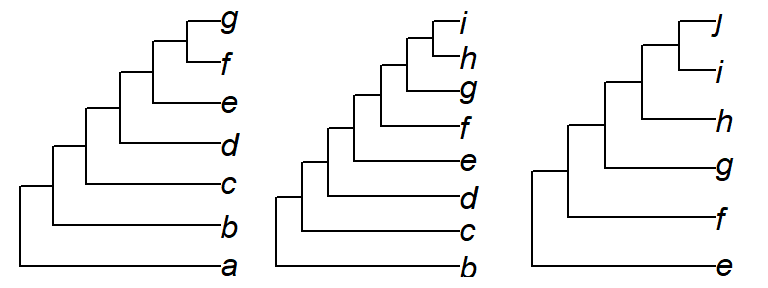This document should contain all you need to get started measuring tree distances with ‘Quartet’. If you get stuck, please let me know so I can improve this documentation.

Instructions for loading phylogenetic trees into R can be found in a separate vignette. For these examples, we’ll enter two simple trees by hand:

tree1 <- ape::read.tree(text = '(A, ((B, (C, (D, E))), ((F, G), (H, I))));')
tree2 <- ape::read.tree(text = '(A, ((B, (C, (D, (H, I)))), ((F, G), E)));')

## Calculating distances

We can calculate distances between pairs of trees using the ‘Quartet’ package.

First we’ll install the package. We can either install the stable version from the CRAN repository:

install.packages('Quartet')

or the development version, from GitHub – which will contain the latest features but may not be as extensively tested:

devtools::install_github('ms609/Quartet')

Then we’ll load the package into R’s working environment:

library('Quartet')

Now the package’s functions are available within R. Let’s proceed to calculate some tree distances.

### Pairs of trees

Calculating the distance between two trees is a two stage process. For a quartet distance, we first have to calculate the status of each quartet:

statuses <- QuartetStatus(tree1, tree2)

Then we convert these counts into a distance metric (or similarity measure) that suits our needs – perhaps the Quartet Divergence:

QuartetDivergence(statuses, similarity = FALSE)
##  0.6031746

We can calculate all similarity metrics at once using:

SimilarityMetrics(statuses, similarity = TRUE)
##      DoNotConflict ExplicitlyAgree StrictJointAssertions
## [1,]     0.3968254       0.3968254             0.3968254
##      SemiStrictJointAssertions SymmetricDifference MarczewskiSteinhaus
## [1,]                 0.3968254           0.3968254           0.2475248
##      SteelPenny QuartetDivergence SimilarityToReference
## [1,]  0.3968254         0.3968254             0.3968254

It can be instructive to visualize how each split in the tree is contributing to the quartet similarity:

VisualizeQuartets(tree1, tree2)Rather than using quartets, we might want to use partitions as the basis of our comparison:

SimilarityMetrics(SplitStatus(tree1, tree2))
##      DoNotConflict ExplicitlyAgree StrictJointAssertions
## [1,]     0.3333333       0.3333333             0.3333333
##      SemiStrictJointAssertions SymmetricDifference MarczewskiSteinhaus
## [1,]                 0.3333333           0.3333333                 0.2
##      SteelPenny QuartetDivergence SimilarityToReference
## [1,]  0.3333333         0.3333333             0.3333333

### Multiple comparisons

If you have more than two trees to compare, you can send a list of trees (class: list or multiPhylo) to the distance comparison function.

You can calculate the similarity between one tree and a forest of other trees:

library('TreeTools', quietly = TRUE, warn.conflicts = FALSE)
oneTree <- CollapseNode(as.phylo(0, 11), 14)
twoTrees <- structure(list(bal = BalancedTree(11), pec = PectinateTree(11)),
class = 'multiPhylo')

status <- SharedQuartetStatus(twoTrees, cf = oneTree)
QuartetDivergence(status)
##       bal       pec
## 0.4939394 0.6272727

Or between one tree and (itself and) all other trees in the forest:

forest <- as.phylo(0:5, 11)
names(forest) <- letters[1:6]
status <- SharedQuartetStatus(forest)
QuartetDivergence(status)
##         a         b         c         d         e         f
## 1.0000000 0.9757576 0.9757576 0.9333333 0.9121212 0.9333333

Or between each pair of trees in a forest:

status <- ManyToManyQuartetAgreement(forest)
QuartetDivergence(status, similarity = FALSE)
##            a          b          c          d          e          f
## a 0.00000000 0.02424242 0.02424242 0.06666667 0.08787879 0.06666667
## b 0.02424242 0.00000000 0.02424242 0.08787879 0.06666667 0.06666667
## c 0.02424242 0.02424242 0.00000000 0.08484848 0.08484848 0.04242424
## d 0.06666667 0.08787879 0.08484848 0.00000000 0.04242424 0.04242424
## e 0.08787879 0.06666667 0.08484848 0.04242424 0.00000000 0.04242424
## f 0.06666667 0.06666667 0.04242424 0.04242424 0.04242424 0.00000000

Or between one list of trees and a second:

status <- TwoListQuartetAgreement(forest[1:4], forest[5:6])
QuartetDivergence(status, similarity = FALSE)
##            e          f
## a 0.08787879 0.06666667
## b 0.06666667 0.06666667
## c 0.08484848 0.04242424
## d 0.04242424 0.04242424

## Trees with different tip labels

“Quartet” can compare trees of different sizes or with non-identical sets of taxa. Quartets pertaining to a leaf that does not occur in one tree are treated as unresolved.

treeAG <- PectinateTree(letters[1:7])
treeBI <- PectinateTree(letters[2:9])
treeEJ <- PectinateTree(letters[5:10])
par(mfrow = c(1, 3), mar = rep(0.3, 4), cex = 1)
plot(treeAG); plot(treeBI); plot(treeEJ)QuartetState(letters[1:4], treeAG) # 3: C is closest to D
##  3
QuartetState(letters[1:4], treeBI) # 0: unresolved in this tree
##  0
# Calculate status for all leaves observed in trees: here, A..I
QuartetStatus(treeAG, treeBI, nTip = TRUE)
##        N   Q  s d r1 r2  u
## [1,] 252 126 15 0 20 55 36
# Calculate status for specified number of leaves
# Here, we have ten taxa A..J, but J does not occur in either of these trees
QuartetStatus(treeAG, treeBI, nTip = 10)
##        N   Q  s d r1 r2   u
## [1,] 420 210 15 0 20 55 120
# Compare a list of trees with different numbers of leaves to a reference
QuartetStatus(c(treeAG, treeBI, treeEJ), cf = treeAG, nTip = TRUE)
##        N   Q  s d r1 r2   u
## [1,] 420 210 35 0  0  0 175
## [2,] 420 210 15 0 55 20 120
## [3,] 420 210  0 0 15 35 160
# Compare all pairs of trees in a list.
# "u" shows how many possible quartets are unresolved in both trees
ManyToManyQuartetAgreement(c(treeAG, treeBI, treeEJ), nTip = TRUE)[, , "u"]
##      [,1] [,2] [,3]
## [1,]  175  120  160
## [2,]  120  140  130
## [3,]  160  130  195

## Other calculations

To calculate how many quartets are unique to a certain tree (akin to the partitionwise equivalent ape::prop.clades), use:

interestingTree <- as.phylo(42, 7)
referenceTrees <- list(BalancedTree(7), PectinateTree(7))
status <- CompareQuartetsMulti(interestingTree, referenceTrees)

status['x_only'] = 23 quartets are resolved in a certain way in interestingTree, but not resolved that way in any referenceTrees.

You may wish to: